HoldPattern

You might be very surprised that the following evaluates to True!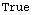When the cell above is evaluated  Times[p__] evaluates to (p__) before the kernel checks to see if the pattern matches.  MatchQ then determines if And[x,y,z] matches the pattern (p__) and it does because And[x,y,z] is itself a sequence of one.

Now the next cell also evaluates to True because both ( And[p__] ) and ( Times[p__] ) evaluate to ( p__ ).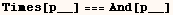We can prevent patterns in the examples above from evaluating by wrapping them with HoldPattern as in the following lines.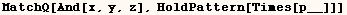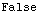With the next line one might expect to have the head (And) changed to (List), but it doesn't work.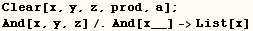In the next cell HoldPattern is used to ensure the head (And) is changed to (List).  The two examples that follow have the same effect, but the use of HoldPattern isn't needed.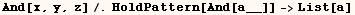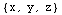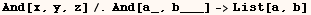HoldPattern wasn't needed in the last example because ( And[a_,b__] ) evaluates to itself.

Sometimes we need to use HoldPattern with care.  Suppose we wanted to replace a product of terms to the (1/3) power with (prod).  We can attempt to do this on (expr) below.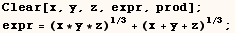In the next line HoldPattern isn't used, andevaluates to.  Then both terms of expr match the evaluated pattern.  We then get (prod+prod)→(2 prod).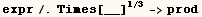Now if the entire pattern is wrapped in HoldPattern, the exponent in the pattern is held in the form
(Times[1,Power[3,-1]] )  instead of  ( Rational[1,3] ), and neither term matches the pattern.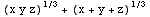The desired result is given when the expression HoldPattern[Times[__]] is raised to the (1/3) power.An easier way to get the desired result is to use  (_Times)^(1/3)  as a pattern.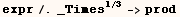Alan Hayes explained that HoldPattern[_] is also needed to ensure that the rule below is used.  Similar to the other examples HoldPattern is needed because Dt[x_] evaluates to something other than itself.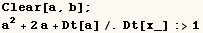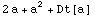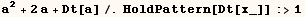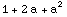Created by Mathematica  (May 16, 2004)

Back to Ted’s Tricks index page